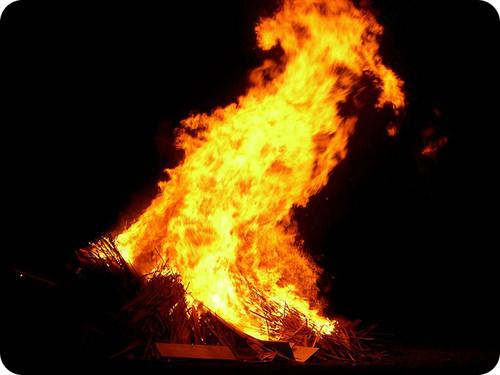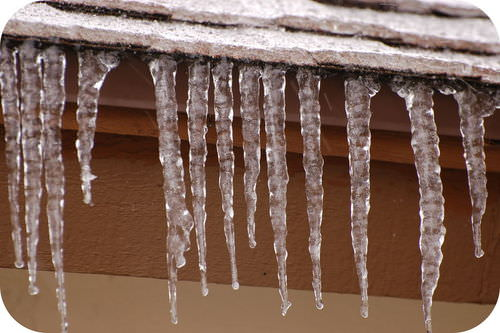# 11.5: Spontaneous Reactions and Free Energy

•• Contributed by Allison Soult
• Senior Lecturer (Chemistry) at University of Kentucky

Learning Outcomes

• Describe the meaning of a spontaneous reaction in terms of enthalpy and entropy changes.
• Define free energy.
• Determine the spontaneity of a reaction based on the value of its change in free energy at high and low temperatures.

The change in enthalpy and change in entropy of a reaction are the driving forces behind all chemical reactions. In this lesson, we will examine a new function called free energy, which combines enthalpy and entropy and can be used to determine whether or not a given reaction will occur spontaneously.

## Spontaneous Reactions

A spontaneous reaction is a reaction that favors the formation of products at the conditions under which the reaction is occurring. A roaring bonfire (see figure below) is an example of a spontaneous reaction. A fire is exothermic, which means a decrease in the energy of the system as energy is released to the surroundings as heat. The products of a fire are composed mostly of gases such as carbon dioxide and water vapor, so the entropy of the system increases during most combustion reactions. This combination of a decrease in energy and an increase in entropy means that combustion reactions occur spontaneously.Figure $$\PageIndex{1}$$: Combustion reactions, such as this fire, are spontaneous reactions. Once the reaction begins, it continues on its own until one of the reactants (fuel or oxygen) is gone.

A nonspontaneous reaction is a reaction that does not favor the formation of products at the given set of conditions. In order for a reaction to be nonspontaneous, one or both of the driving forces must favor the reactants over the products. In other words, the reaction is endothermic, is accompanied by a decrease in entropy, or both. Out atmosphere is composed primarily of a mixture of nitrogen and oxygen gases. One could write an equation showing these gases undergoing a chemical reaction to form nitrogen monoxide.

$\ce{N_2} \left( g \right) + \ce{O_2} \left( g \right) \rightarrow 2 \ce{NO} \left( g \right)$

Fortunately, this reaction is nonspontaneous at normal temperatures and pressures. It is a highly endothermic reaction with a slightly positive entropy change $$\left( \Delta S \right)$$. However, nitrogen monoxide is capable of being produced at very high temperatures, and this reaction has been observed to occur as a result of lightning strikes.

One must be careful not to confuse the term spontaneous with the notion that a reaction occurs rapidly. A spontaneous reaction is one in which product formation is favored, even if the reaction is extremely slow. You do not have to worry about a piece of paper on your desk suddenly bursting into flames, although its combustion is a spontaneous reaction. What is missing is the required activation energy to get the reaction started. If the paper were to be heated to a high enough temperature, it would begin to burn, at which point the reaction would proceed spontaneously until completion.

In a reversible reaction, one reaction direction may be favored over the other. Carbonic acid is present in carbonated beverages. It decomposes spontaneously to carbon dioxide and water according to the following reaction.

$\ce{H_2CO_3} \left( aq \right) \rightleftharpoons \ce{CO_2} \left( g \right) + \ce{H_2O} \left( l \right)$

If you were to start with pure carbonic acid in water and allow the system to come to equilibrium, more than $$99\%$$ of the carbonic acid would be converted into carbon dioxide and water. The forward reaction is spontaneous because the products of the forward reaction are favored at equilibrium. In the reverse reaction, carbon dioxide and water are the reactants, and carbonic acid is the product. When carbon dioxide is bubbled into water (see figure below), less than $$1\%$$ is converted to carbonic acid when the reaction reaches equilibrium. The reverse of the above reaction is not spontaneous. This illustrates another important point about spontaneity. Just because a reaction is not spontaneous does not mean that it does not occur at all. Rather, it means that the reactants will be favored over the products at equilibrium, even though some products may indeed form.Figure $$\PageIndex{2}$$: A home soda making machine is shown with a bottle of water and a $$\ce{CO_2}$$ cartridge. When the water is carbonated, only a small amount of carbonic acid is formed because the reaction is nonspontaneous. (Public Domain; Baruchlanda)

## Gibbs Free Energy

Many chemical reactions and physical processes release energy that can be used to do other things. When the fuel in a car is burned, some of the released energy is used to power the vehicle. Free energy is energy that is available to do work. Spontaneous reactions release free energy as they proceed. Recall that the determining factors for spontaneity of a reaction are the enthalpy and entropy changes that occur for the system. The free energy change of a reaction is a mathematical combination of the enthalpy change and the entropy change.

$\Delta G^\text{o} = \Delta H^\text{o} - T \Delta S^\text{o}$

The symbol for free energy is $$G$$, in honor of American scientist Josiah Gibbs (1839 - 1903), who made many contributions to thermodynamics. The change in Gibbs free energy is equal to the change in enthalpy minus the mathematical product of the change in entropy multiplied by the Kelvin temperature. Each thermodynamic quantity in the equation is for substances in their standard states, as indicated by the $$^\text{o}$$ superscripts.

A spontaneous reaction is one that releases free energy, and so the sign of $$\Delta G$$ must be negative. Since both $$\Delta H$$ and $$\Delta S$$ can be either positive or negative, depending on the characteristics of the particular reaction, there are four different possible combinations. The outcomes for $$\Delta G$$ based on the signs of $$\Delta H$$ and $$\Delta S$$ are outlined in the table below. Recall that $$- \Delta \text{H}$$ indicates that the reaction is exothermic and a $$+ \Delta \text{H}$$ means the reaction is endothermic. For entropy, $$+ \Delta \text{S}$$ means the entropy is increasing and the system is becoming more disordered. A $$- \Delta \text{S}$$ means that entropy is decreasing and the system is becoming less disordered (more ordered).

Table $$\PageIndex{1}$$: Enthalpy, Entropy, and Free Energy Changes.
$$\Delta H$$ $$\Delta S$$ $$\Delta G$$
negative positive always negative
positive positive negative at higher temperatures, positive at lower temperatures
negative negative negative at lower temperatures, positive at higher temperatures
positive negative always positive

Keep in mind that the temperature in the Gibbs free energy equation is the Kelvin temperature, so it can only have a positive value. When $$\Delta H$$ is negative and $$\Delta S$$ is positive, the sign of $$\Delta G$$ will always be negative, and the reaction will be spontaneous at all temperatures. This corresponds to both driving forces being in favor of product formation. When $$\Delta H$$ is positive and $$\Delta S$$ is negative, the sign of $$\Delta G$$ will always be positive, and the reaction can never be spontaneous. This corresponds to both driving forces working against product formation.

When one driving force favors the reaction, but the other does not, it is the temperature that determines the sign of $$\Delta G$$. Consider first an endothermic reaction (positive $$\Delta H$$) that also displays an increase in entropy (positive $$\Delta S$$). It is the entropy term that favors the reaction. Therefore, as the temperature increases, the $$T \Delta S$$ term in the Gibbs free energy equation will begin to predominate and $$\Delta G$$ will become negative. A common example of a process which falls into this category is the melting of ice (see figure below). At a relatively low temperature (below $$273 \: \text{K}$$), the melting is not spontaneous because the positive $$\Delta H$$ term "outweighs" the $$T \Delta S$$ term. When the temperature rises above $$273 \: \text{K}$$, the process becomes spontaneous because the larger $$T$$ value has tipped the sign of $$\Delta G$$ over to being negative.Figure $$\PageIndex{3}$$: Ice melts spontaneously only when the temperature is above $$0^\text{o} \text{C}$$. The increase in entropy is then able to drive the unfavorable endothermic process.

When the reaction is exothermic (negative $$\Delta H$$) but undergoes a decrease in entropy (negative $$\Delta S$$), it is the enthalpy term which favors the reaction. In this case, a spontaneous reaction is dependent upon the $$T \Delta S$$ term being small relative to the $$\Delta H$$ term, so that $$\Delta G$$ is negative. The freezing of water is an example of this type of process. It is spontaneous only at a relatively low temperature. Above $$273. \: \text{K}$$, the larger $$T \Delta S$$ value causes the sign of $$\Delta G$$ to be positive, and freezing does not occur.